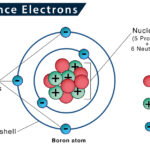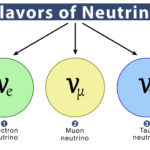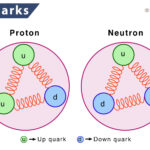Home / Chemistry / Electron Configuration

# Electron Configuration

The electron configuration describes the position of electrons of an atom or a molecule in atomic or molecular orbitals. It is a type of code that shows the number of electrons in each atom’s energy level and how they are arranged within each energy level.

For each electron, there is a ground state and an excited state. Here, we will discuss the ground state electron configuration, which is about the electron configuration of an atom at the lowest possible energy level. The other state, the excited state, means that the valence electron has moved from its ground state orbital to another higher energy orbital.

Based on the electronic configuration, the chemical reactivity of substances can be predicted, what kind of reaction is likely to happen and how strong the reaction will be. The electron configuration is also helpful in determining the valency of an element.

## How to Write Electron Configurations with Periodic Table

The electron configuration is written using a standard notation, where all electron-containing atomic sub-shells are placed in a sequence of their filling order.

The periodic table helps find the electron configurations of the elements. The principle energy level is represented by an integer (1, 2, 3, …7) corresponding to the periods on the periodic table. Each successive integer typically represents a higher energy level than the last. Letters like s, p, d, and f are the subshells.

Given below is the complete electron configuration of the hydrogen element.

The first integer, 1, represents the principle energy level, the letter ‘s’ represents the type of orbital (sublevel), and the superscript 1 gives the electron occupancy. Here, only one electron in the s orbital has the principle energy level of 1.

Systems having a large number of electrons will occupy greater energy levels. The electron configuration of potassium (K) is 1s22s22p63s23p64s1. Here, 1s, 2s, 3s, 3p, and 4s represent the sub-shells, whereas their superscripts denote the number of electrons in each sub-shell. Similarly, the configuration of phosphorous, located in the third period and within the p-block, is 1s22s22p63s23p3.

### Abbreviated Notation (Shorthand Method)

However, the standard notation often gives lengthy electron configurations, mainly for elements having a relatively large atomic number. In those elements, an abbreviated or condensed notation is used. Here, the sequence of filled sub-shells corresponding to the electronic configuration of its closest noble gas is replaced with the symbol of that noble gas using square brackets.

Let us consider the element potassium (K), written using its nearest noble gas configuration, i.e., argon. The electron configuration of argon is 1s22s22p63s23p6, and thus the abbreviated notation of potassium is written as [Ar] 4s1. Similarly, the electronic configuration of argon is written using its nearest inert neighbor neon, having the electronic configuration 1s22s22p6. So, the abbreviated notation for argon would be [Ne] 3s²3p⁶.

### Electron Configuration of Some Other Common Elements

Oxygen

The atomic number of oxygen is 8. It implies that an oxygen atom has 8 electrons, filled in the following order: 2 (in K shell) and 6 (in L shell).

Therefore, the electron configuration of oxygen is 1s22s22p4 or [He] 2s²2p4.

Chlorine

Chlorine has the atomic number 17. Its 17 electrons are distributed as follows: 2 (in K shell), 8 (in L shell), and 7 (in M shell).

Thus, the electron configuration of chlorine can be given as 1s22s22p63s23p5 or as [Ne] 3s23p5.

## Rules for Filling Atomic Orbitals in Order

As discussed, the number of electrons in each atomic orbital of every sub-shell determines the electronic configuration of that particular atom. However, the electrons follow some general rules while entering an orbital.

### Aufbau Principle

Aufbau means “building up” in German, so this rule states that electrons will occupy the lower energy orbitals before occupying those with higher energy. Thus, according to this, principal electrons are filled in the order: 1s -> 2s -> 2p -> 3s -> 3p -> 4s -> 3d -> 4p -> 5s -> 4d -> 5p -> 6s -> 4f -> 5d -> 6p -> 7s -> 5f -> 6d -> 7p and so on.

Whereas the energy level of an orbital is calculated by the sum of the principal (n) quantum number and the azimuthal (l) quantum numbers, written as n+l. In the case of an equal ‘n+l’ value, the orbitals having a lower ‘n’ value are filled first.

However, exceptions exist to the Aufbau principle, such as chromium and copper, explained by the stability provided by half-filled or completely filled sub-shells.

### Pauli-Exclusion Principle

According to this rule, a maximum of two electrons, each having opposite spins, can fit in an orbital. In another way, we can say no two electrons in the same atom can have the same values for all four quantum numbers. Thus, if the principal, azimuthal, and magnetic numbers are the same for two electrons, they must have opposite spins.

### Hund’s Rule

It states that electrons singly occupy every orbital in a given sub-shell before a second electron occupies its space. Hund’s rule describes the order in which electrons are filled in all the orbitals belonging to a sub-shell.

To maximize the total spin, the electrons in the orbitals that contain only one electron all possess the same spin, i.e., the exact values of the spin quantum number.

## Electron Configuration and Orbital Diagrams

The other way to represent an electron configuration is through an orbital diagram. In an orbital diagram, orbitals are given as boxes, and electrons are represented by arrows (↑ or ↓), with two electrons occupying each box. Orbitals are labeled based on their principal energy levels and sublevels (1s, 2p, and so on).

Listed below are the orbital diagrams of a few more common elements, along with their electron configuration:Valence ElectronsGluonNeutrinoQuark